# Number plate recognition with Tensorflow

## Introduction

Over the past few weeks I’ve been dabbling with deep learning, in particular convolutional neural networks. One standout paper from recent times is Google’s Multi-digit Number Recognition from Street View. This paper describes a system for extracting house numbers from street view imagery using a single end-to-end neural network. The authors then go on to explain how the same network can be applied to breaking Google’s own CAPTCHA system with human-level accuracy.

In order to get some hands-on experience with implementing neural networks I decided I’d design a system to solve a similar problem: Automated number plate recognition (automated license plate recognition if you’re in the US). My reasons for doing this are three-fold:

• I should be able to use the same (or a similar) network architecture as the Google paper: The Google architecture was shown to work equally well at solving CAPTCHAs, as such it’s reasonable to assume that it’d perform well on reading number plates too. Having a known good network architecture will greatly simplify things as I learn the ropes of CNNs.

• I can easily generate training data. One of the major issues with training neural networks is the requirement for lots of labelled training data. Hundreds of thousands of labelled training images are often required to properly train a network. Fortunately, the relevant uniformity of UK number plates means I can synthesize training data.

• Curiosity. Traditional ANPR systems have relied on hand-written algorithms for plate localization, normalization, segmentation, character recognition etc. As such these systems tend to be many thousands of lines long. It’d be interesting to see how good a system I can develop with minimal domain-specific knowledge with a relatively small amount of code.

For this project I’ve used Python, TensorFlow, OpenCV and NumPy. Source code is available here.

## Inputs, outputs and windowing

In order to simplify generating training images and to reduce computational requirements I decided my network would operate on 128x64 grayscale input images.

128x64 was chosen as the input resolution as this is small enough to permit training in a reasonable amount of time with modest resources, but also large enough for number plates to be somewhat readable: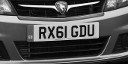Image credit

In order to detect number plates in larger images a sliding window approach is used at various scales: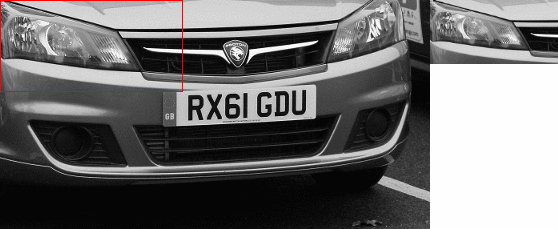Image credit

The image on the right is the 128x64 input that the neural net sees, whereas the left shows the window in the context of the original input image.

For each window the network should output:

• The probability a number plate is present in the input image. (Shown as a green box in the above animation).

• The probability of the digit in each position, ie. for each of the 7 possible positions it should return a probability distribution across the 36 possible characters. (For this project I assume number plates have exactly 7 characters, as is the case with most UK number plates).

A plate is considered present if and only if:

• The plate falls entirely within the image bounds.

• The plate’s width is less than 80% of the image’s width, and the plate’s height is less than 87.5% of the image’s height.

• The plate’s width is greater than 60% of the image’s width or the plate’s height is greater than 60% of the image’s height.

With these numbers we can use a sliding window that moves 8 pixels at a time, and zooms in $\sqrt[] 2$ times between zoom levels and be guaranteed not to miss any plates, while at the same time not generating an excessive number of matches for any single plate. Any duplicates that do occur are combined in a post-processing step (explained later).

## Synthesizing images

To train any neural net a set of training data along with correct outputs must be provided. In this case this will be a set of 128x64 images along with the expected output. Here’s an illustrative sample of training data generated for this project:

•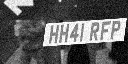expected output HH41RFP 1.
•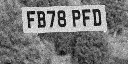expected output FB78PFD 1.
•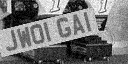expected output JW01GAI 0. (Plate partially truncated.)
•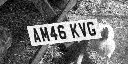expected output AM46KVG 0. (Plate too small.)
•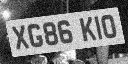expected output XG86KIO 0. (Plate too big.)
•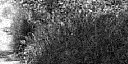expected output XH07NYO 0. (Plate not present at all.)

The first part of the expected output is the number the net should output. The second part is the “presence” value that the net should ouput. For data labelled as not present I’ve included an explanation in brackets.

The process for generating the images is illustrated below:The text and plate colour are chosen randomly, but the text must be a certain amount darker than the plate. This is to simulate real-world lighting variation. Noise is added at the end not only to account for actual sensor noise, but also to avoid the network depending too much on sharply defined edges as would be seen with an out-of-focus input image.

Having a background is important as it means the network must learn to identify the bounds of the number plate without “cheating”: Were a black background used for example, the network may learn to identify plate location based on non-blackness, which would clearly not work with real pictures of cars.

The backgrounds are sourced from the SUN database, which contains over 100,000 images. It’s important the number of images is large to avoid the network “memorizing” background images.

The transformation applied to the plate (and its mask) is an affine transformation based on a random roll, pitch, yaw, translation, and scale. The range allowed for each parameter was selected according to the ranges that number plates are likely to be seen. For example, yaw is allowed to vary a lot more than roll (you’re more likely to see a car turning a corner, than on its side).

The code to generate the images is relatively short (~300 lines). It can be read in gen.py.

## The network

Here’s the network architecture used:See the wikipedia page for a summary of CNN building blocks. The above network is in fact based on this paper by Stark et al, as it gives more specifics about the architecture used than the Google paper.

The output layer has one node (shown on the left) which is used as the presence indicator. The rest encode the probability of a particular number plate: Each column as shown in the diagram corresponds with one of the digits in the number plate, and each node gives the probability of the corresponding character being present. For example, the node in column 2 row 3 gives the probability that the second digit is a C.

As is standard with deep neural nets all but the output layers use ReLU activation. The presence node has sigmoid activation as is typically used for binary outputs. The other output nodes use softmax across characters (ie. so that the probability in each column sums to one) which is the standard approach for modelling discrete probability distributions.

The code defining the network is in model.py.

The loss function is defined in terms of the cross-entropy between the label and the network output. For numerical stability the activation functions of the final layer are rolled into the cross-entropy calculation using softmax_cross_entropy_with_logits and sigmoid_cross_entropy_with_logits. For a detailed and intuitive introduction to cross-entropy see this section in Michael A. Nielsen’s free online book.

Training (train.py) takes about 6 hours using a nVidia GTX 970, with training data being generated on-the-fly by a background process on the CPU.

## Output Processing

To actually detect and recognize number plates in an input image a network much like the above is applied to 128x64 windows at various positions and scales, as described in the windowing section.

The network differs from the one used in training in that the last two layers are convolutional rather than fully connected, and the input image can be any size rather than 128x64. The idea is that the whole image at a particular scale can be fed into this network which yields an image with a presence / character probability values at each “pixel”. The idea here is that adjacent windows will share many convolutional features, so rolling them into the same network avoids calculating the same features multiple times.

Visualizing the “presence” portion of the output yields something like the following: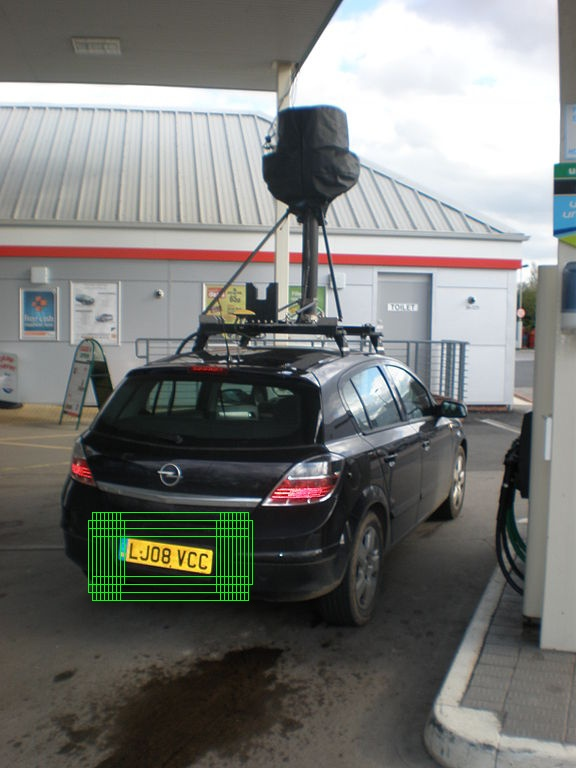Image credit

The boxes here are regions where the network detects a greater than 99% probability that a number plate is present. The reason for the high threshold is to account for a bias introduced in training: About half of the training images contained a number plate, whereas in real world images of cars number plates are much rarer. As such if a 50% threshold is used the detector is prone to false positives.

To cope with the obvious duplicates we apply a form of non-maximum suppression to the output: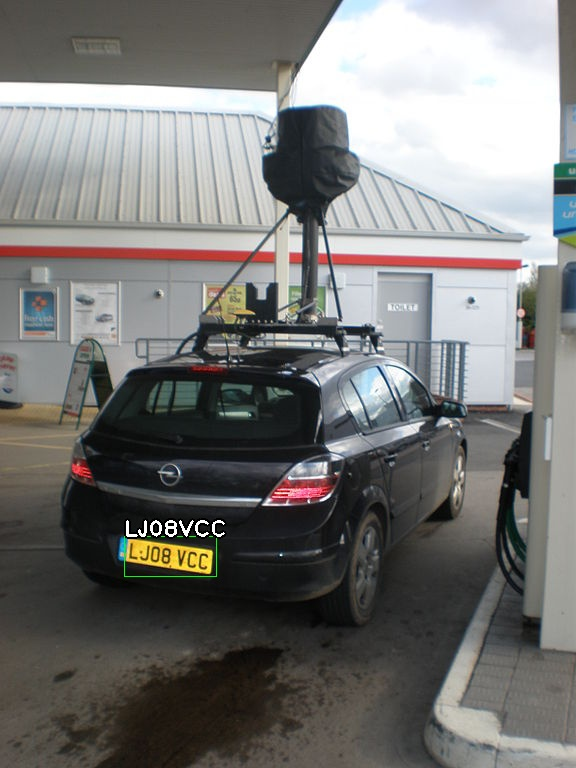Image credit

The technique used here first groups the rectangles into overlapping rectangles, and for each group outputs:

• The intersection of all the bounding boxes.
• The license number corresponding with the box in the group that had the highest probability of being present.

Here’s the detector applied to the image at the top of this post: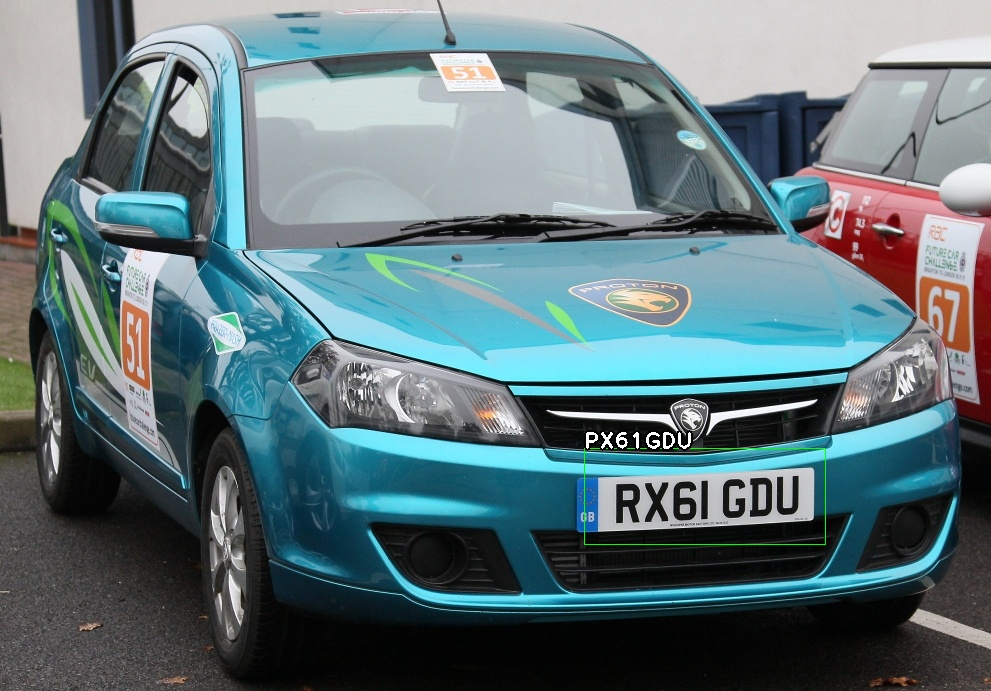Image credit

Whoops, the R has been misread as a P. Here’s the window from the above image which gives the maximum presence response: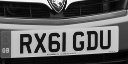Image credit

On first glance it appears that this should be an easy case for the detector, however it turns out to be an instance of overfitting. Here’s the R from the number plate font used to generate the training images: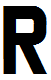Note how the leg of the R is at a different angle to the leg of the R in the input image. The network has only ever seen R’s as shown above, so gets confused when it sees R’s in a different font. To test this hypothesis I modified the image in GIMP to more closely resemble the training font: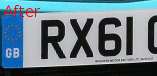And sure enough, the detector now gets the correct result: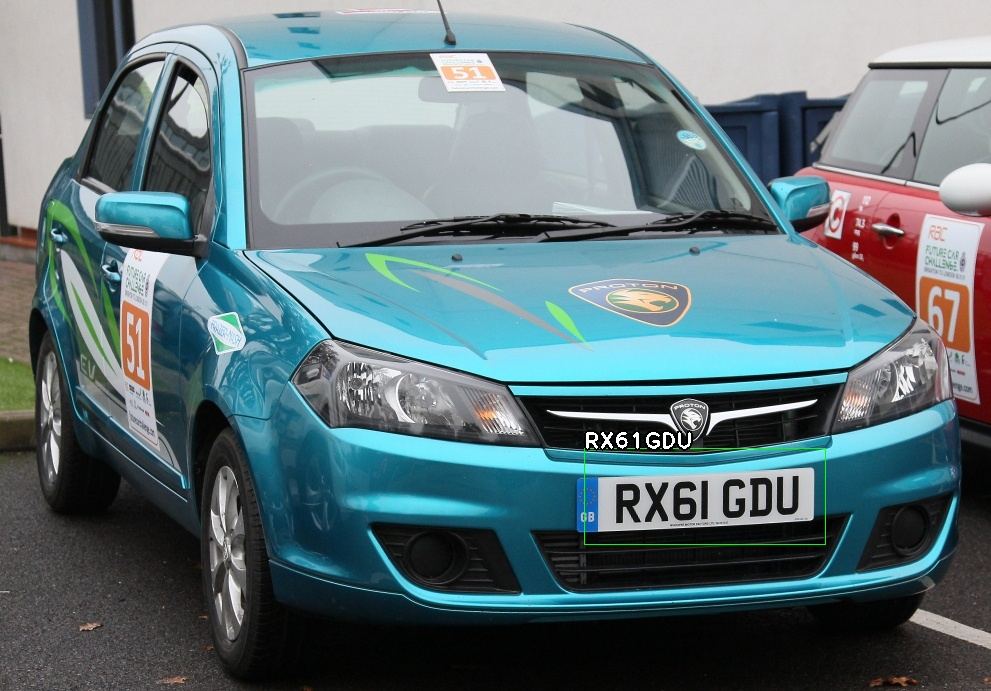The code for the detector is in detect.py.

## Conclusion

I’ve shown that with a relatively short amount of code (~800 lines), its possible to build an ANPR system without importing any domain-specific libraries, and with very little domain-specific knowledge. Furthermore I’ve side-stepped the problem of needing thousands of training images (as is usually the case with deep neural networks) by synthesizing images on the fly.

On the other hand, my system has a number of drawbacks:

1. It only works with number plates in a specific format. More specifically, the network architecture assumes exactly 7 chars are visible in the output.

2. It only works on specific number plate fonts.

3. It’s slow. The system takes several seconds to run on moderately sized image.

The Google team solves 1) by splitting the higher levels of their network into different sub-networks, each one assuming a different number of digits in the output. A parallel sub-network then decides how many digits are present. I suspect this approach would work here, however I’ve not implemented it for this project.

I showed an instance of 2) above, with the misdetection of an R due to a slightly varied font. The effects would be further exacerbated if I were trying to detect US number plates rather than UK number plates which have much more varied fonts. One possible solution would be to make my training data more varied by drawing from a selection of fonts, although it’s not clear how many fonts I would need for this approach to be successful.

The slowness (3)) is a killer for many applications: A modestly sized input image takes a few seconds to process on a reasonably powerful GPU. I don’t think its possible to get away from this without introducing a (cascade of) detection stages, for example a Haar cascade, a HOG detector, or a simpler neural net.

It would be an interesting exercise to see how other ML techniques compare, in particular pose regression (with the pose being an affine transformation corresponding with 3 corners of the plate) looks promising. A much more basic classification stage could then be tacked on the end. This solution should be similarly terse if an ML library such as scikit-learn is used.

In conclusion, I’ve shown that a single CNN (with some filtering) can be used as a passable number plate detector / recognizer, however it does not yet compete with the traditional hand-crafted (but more verbose) pipelines in terms of performance.